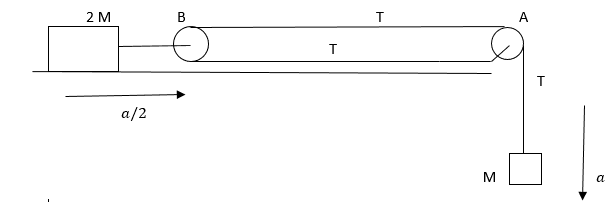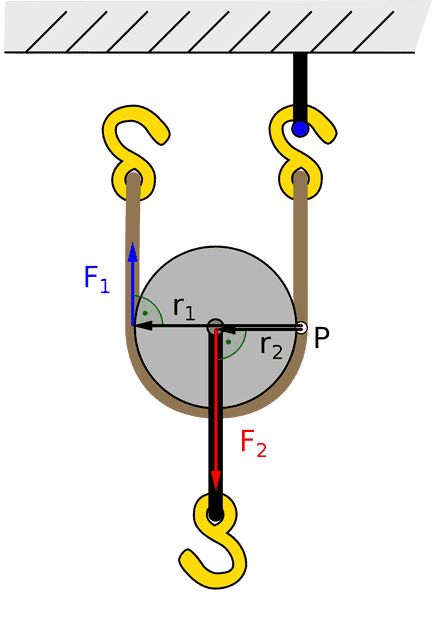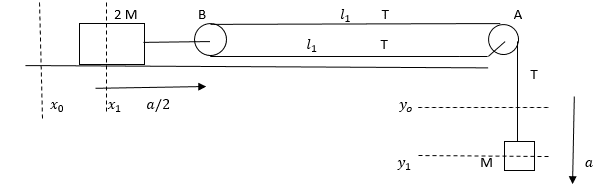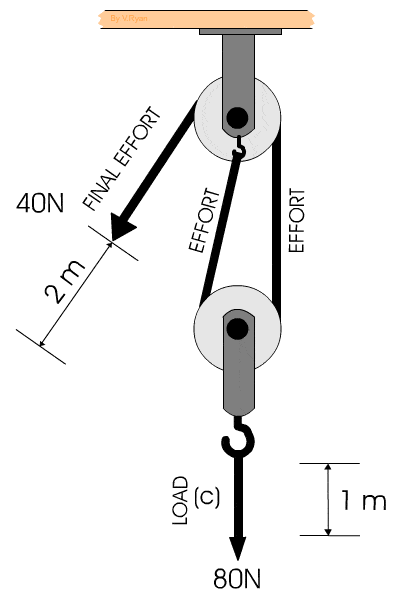# Find the acceleration of the masses

Monsterboy
Homework Statement:
Consider the situation shown in figure. Both the pulleys and the string are light and all the surfaces are friction-less. Find the acceleration of the mass M and the tension in the string in the
figure given below.
Relevant Equations:
Free-body diagrams and the forces acting on them.I solved this problem by assuming that the acceleration ##a## is the same on the both the masses M and 2M but then answers were wrong, but if the acceleration of the mass 2M is considered as ##a/2## then I get the right answers, but I don't how exactly acceleration is getting halved for mass 2M.

How do I show that the acceleration of the mass 2M is ##a/2## if the acceleration of M is ##a## ?

Homework Helper
Gold Member
Can you visualize pulley B as being a lever with pivot located at the lowest point, load located at the middle point and working force located at the highest point?
Then, combine that idea with the mechanical advantage concept: what you gain in force, you lose in displacement.•Monsterboy
Homework Helper
Gold Member
2022 Award
Homework Statement:: Consider the situation shown in figure. Both the pulleys and the string are light and all the surfaces are friction-less. Find the acceleration of the mass M and the tension in the string in the
figure given below.
Relevant Equations:: Free-body diagrams and the forces acting on them.

How do I show that the acceleration of the mass 2M is a/2 if the acceleration of M is a ?
By using the fact that the string has constant total length.
Write an expression for the length of the string in terms of the locations of the masses and differentiate it wrt time a couple of times.

•Monsterboy and Lnewqban
Homework Helper
Gold Member
2022 Award
Homework Statement:: Consider the situation shown in figure. Both the pulleys and the string are light and all the surfaces are friction-less. Find the acceleration of the mass M and the tension in the string in the
figure given below.
Relevant Equations:: Free-body diagrams and the forces acting on them.

View attachment 299117
I solved this problem by assuming that the acceleration ##a## is the same on the both the masses M and 2M but then answers were wrong, but if the acceleration of the mass 2M is considered as ##a/2## then I get the right answers, but I don't how exactly acceleration is getting halved for mass 2M.

How do I show that the acceleration of the mass 2M is ##a/2## if the acceleration of M is ##a## ?
If you move mass ##M## by ##1## unit, then mass ##2M## moves by ##0.5## units. That's a constraint on the motion.

Note that if you pushed mass ##M## up, then mass ##2M## would not move. Again, in that case, an assumption of equal accelerations would be wrong.

•Monsterboy
Monsterboy
By using the fact that the string has constant total length.
Write an expression for the length of the string in terms of the locations of the masses and differentiate it wrt time a couple of times.##{x_0}## and ##{x_1}## are distances from mass 2M to pulley B, they are constants.
##{y_0}## and ##{y_1}## are the distances of mass M from pulley A.
##{l_0}## and ##{l_1}## are the distances between the pulleys A and B with respect to positions ##{x_0}## and ##{x_1}## of mass 2M and ##{y_0}## and ##{y_1}## of mass M respectively.

Let the total length of the string be ##L##

##L = f(y, l)##

##L = 2{l_0} + {y_0}##
##L = 2{l_1} + {y_1}##

## L = 2l + y ##
Differentiating the above with respect to time
## \frac{dL}{dt} = 0 = \frac{2dl}{dt} + \frac{dy}{dt} ##
## \frac{2dl}{dt} + \frac{dy}{dt} = 0 ##
The above means ## 2({l_1} - {l_0}) = {y_1} - {y_0} ## in the time interval ##t## right ?

Last edited:
Homework Helper
Gold Member
2022 Award
That's essentially correct, but can you see directly that if the mass ##M## moves down by a distance ##\Delta y##, then the distance between the pulleys reduces by ##\frac {\Delta y}{2}##?

Monsterboy
That's essentially correct, but can you see directly that if the mass ##M## moves down by a distance ##\Delta y##, then the distance between the pulleys reduces by ##\frac {\Delta y}{2}##?
Some people told me that but I am not able to visualize the distance moved by the pulley B to be exactly equal to ##\frac {\Delta y}{2}##

Homework Helper
Gold Member
2022 Award
View attachment 299169

##{x_0}## and ##{x_1}## are distances from mass 2M to pulley B, they are constants.
##{y_0}## and ##{y_1}## are the distances of mass M from pulley A.
##{l_0}## and ##{l_1}## are the distances between the pulleys A and B with respect to positions ##{x_0}## and ##{x_1}## of mass 2M and ##{y_0}## and ##{y_1}## of mass M respectively.

Let the total length of the string be ##L##

##L = f(x, y, l)##

##L = 2{l_0} + {x_0} + {y_0}##
##L = 2{l_1} + {x_1} + {y_1}##

## L = 2l + x + y ##
Differentiating the above with respect to time
## \frac{dL}{dt} = 0 = \frac{2dl}{dt} + 0 + \frac{dy}{dt} ##
## \frac{2dl}{dt} + \frac{dy}{dt} = 0 ##
The above means ## 2({l_1} - {l_0}) = {y_1} - {y_0} ## in the time interval ##t## right ?
Yes, but it’s the accelerations you want, so differentiate a second time.
Some people told me that but I am not able to visualize the distance moved by the pulley B to be exactly equal to ##\frac {\Delta y}{2}##
That's why I suggested this approach. You can use it in quite complicated arrangements without getting confused.

•Monsterboy
Homework Helper
Gold Member
2022 Award
Some people told me that but I am not able to visualize the distance moved by the pulley B to be exactly equal to ##\frac {\Delta y}{2}##
If you can't see something like this, then it's a lot of work and different variables to piece it all together. For eaxmple: the total length of the string, ##l##, is$$l = 2l_{AB} + l_{AM}$$And the position of pulley ##B## is the distance to ##A## from some fixed origin less the length of string ##l_{AB}##:
$$x_B = d_{OA} - l_{AB}$$And the vertical position of mass ##M## we can take to be ##y_M = l_{AM}##. Putting these together we get:
$$x_B = d_{OA} - \frac{l}{2} + \frac{y_M}{2}$$Finally, the distance from mass ##2M## to ##B## is constant, so:
$$x_{2M} = \frac{y_M}{2} + c$$for some constant ##c##, which we can take to be zero by a suitable choice of origin.

That's a lot of effort just to establish that $$x_{2M} = \frac{y_M}{2}$$which I would simply have written down as a system constraint. I wouldn't expect any exam marker to challenge that or expect more.

•Lnewqban
Monsterboy
That's essentially correct, but can you see directly that if the mass ##M## moves down by a distance ##\Delta y##, then the distance between the pulleys reduces by ##\frac {\Delta y}{2}##?
So half of ##\Delta y## of the string moves towards the pulley B from under it and the other half moves away, over it, summing up to ##\Delta y## length of string moving towards right, so the distance moved by the pulley is ##\frac {\Delta y}{2}## is this how you see it ?

•Lnewqban
Homework Helper
Gold Member
2022 Award
So half of ##\Delta y## of the string moves towards the pulley B from under it and the other half moves away, over it, summing up to ##\Delta y## length of string moving towards right, so the distance moved by the pulley is ##\frac {\Delta y}{2}## is this how you see it ?
Yes, the string runs twice between ##A## and ##B##. If ##B## moves ##1 \ cm## closer to ##A##, then you lose ##2 \ cm## of string.

Homework Helper
Gold Member
Some people told me that but I am not able to visualize the distance moved by the pulley B to be exactly equal to ##\frac {\Delta y}{2}##•nasu
•